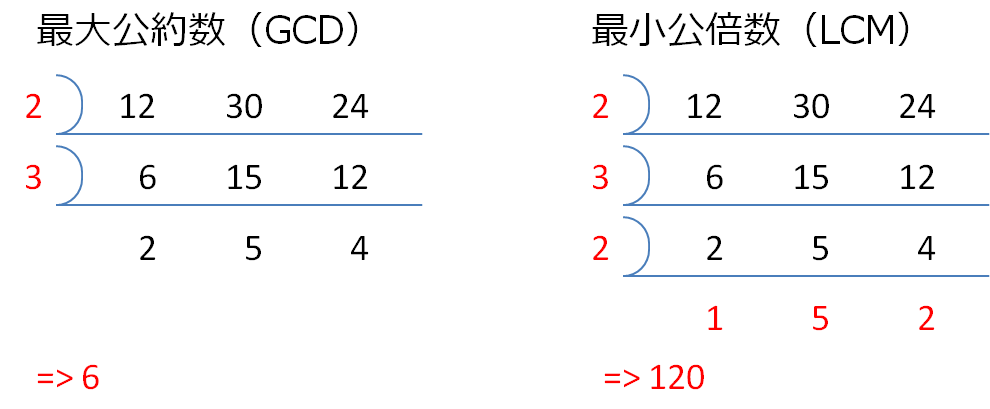# [JAVA] About the continuous division method learned in the 4th grade of elementary school

It has a math tag, but it's math.

# Continuous division method

I learned the greatest common divisor and the least common multiple in the 4th grade of elementary school, but I learned that there is a continuous division method (both blind calculation) in the "how to do" at that time.

## How to do### Greatest common divisor (GCD)

If there are divisors common to a set of integers, reduce them and multiply all the divisors to find them.

### Least common multiple (LCM)

If there are two or more common divisors in a set of integers, reduce them, and multiply the divisor and the remainder to find it.

## Common GCD, LCM

Of course, it can be easily done by Euclidean algorithm. Even if there are more than two pairs of integers, it can be solved with \$ gcd (a, b, c) = gcd (gcd (a, b), c) \$.

``````public int gcd(int a, int b) {
if (b == 0) return a;
return gcd(b, a % b);
}

public int lcm(int a, int b) {
return a * b / gcd(a, b);
}
``````

## Try to reimplement

However, since the purpose is that my daughter can reproduce it in Scratch, I will try to assemble it in Java for the time being. Source

--Try to reduce a set of integers with prime numbers

Since the method of contracting is different between GCD and LCM, Predicate is replaced. You can pass functions in Java too!

``````Integer primeFactory(List<Integer> intList) {
Predicate<Integer> op = mode.equals(Mode.GCD) ? factoryAll(intList) : factoryMulti(intList);
return primeList.stream().filter(op).findFirst().orElse(1);
}

static Predicate<Integer> factoryAll(List<Integer> intList) {
return i -> intList.stream().allMatch(isDivisable(i));
}

static Predicate<Integer> factoryMulti(List<Integer> intList) {
return i -> intList.stream().filter(isDivisable(i)).count() > 1;
}
``````

――If you can make a contract, move on to the next stage

Generate a new set of integers by dividing the original set of integers by a divisor. UnaryOperator is passing unary operations. If it is not divisible by LCM, the original integer is returned.

``````static List<Integer> divideList(Integer divisor, List<Integer> intList) {
return intList.stream().map(divide(divisor)).collect(Collectors.toList());
}

static UnaryOperator<Integer> divide(Integer divisor) {
return i -> (i % divisor) == 0 ? i / divisor : i;
}
``````

--Finally multiply all the objects

``````Integer getGCD() {
return reduceDivisor();
}
Integer getLCM() {
return getLastValue().stream().reduce(1, (x, y) -> x * y) * reduceDivisor();
}
Integer reduceDivisor() {
return stack.stream().map(p -> p.getKey()).reduce(1, (x, y) -> x * y);
}
``````

## Afterword

There are three steps, but it seems to be difficult to build with Scratch, so it is pending. Which is the prime number derivation?